Definitions of Square Dance Calls and Concepts

Index -->  Plus  |  A1  |  A2  |  C1  |  C2  |  C3A  |  C3B  |  C4  |  NOL  |
Definitions (Text Only) -->  Plus  |  A1  |  A2  |  C1  |  C2  |  C3A  |  C3B  |  C4  |  NOL  |
 Find call:

 \$B8@8l(B

Break up the call into its component parts, and do the parts in reverse order. EN: 10
\$BM?\$(\$i\$l\$?%3!<%k\$r9=@.MWAG\$N%Q!<%H\$KJ,2r\$7\$F(B, \$B\$=\$N%Q!<%H\$r5U\$N=g=x\$G9T\$\$\$^\$9(B. JP: 10

\$BCalls With Parts.

Reverse Order Recoil: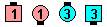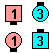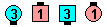Reverse Order Recoil\$B\$NA0(B Step & Fold\$B\$N8e(B Box Recycle\$B\$N8e(B (\$B=*\$o\$j(B)
Reverse Order Travel Thru: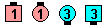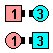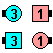Reverse Order Travel Thru\$B\$NA0(B As Couples 1/4 Right\$B\$N8e(B Pass Thru\$B\$N8e(B (\$B=*\$o\$j(B)
Reverse Order Diamond Chain Thru: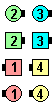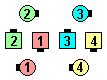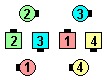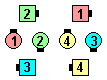Reverse Order Diamond Chain Thru\$B\$NA0(B Centers Cast Off 3/4\$B\$N8e(B Very Centers Trade\$B\$N8e(B Diamond Circulate\$B\$N8e(B (\$B=*\$o\$j(B)

Note: Some callers simply say Reverse when they mean Reverse Order. This can often be ambiguous. Consider Reverse Recoil: does the caller want a Reverse (Mirror) Recycle then Step & Fold, or does the caller want a Step & Fold then Split Recycle? EN: 20
\$BCmReverse Order \$B\$r0UL#\$9\$k\$H\$-\$K\$H\$-\$K(B, \$BC1\$K(B Reverse \$B\$H8@\$\$\$^\$9(B. \$B\$3\$l\$O(B, \$BB?\$/\$N>l9g\$"\$\$\$^\$\$\$G\$9(B. Reverse Recoil \$B\$r9M\$(\$F\$_\$k\$H(B: \$B%3!<%i!<\$O(B, Reverse (Mirror) Recycle \$B\$r\$7\$F(B Step & Fold \$B\$r\$7\$?\$\$\$N\$+(B, \$B\$^\$?\$O(B Step & Fold \$B\$r\$7\$F(B Split Recycle \$B\$r\$7\$?\$\$\$N\$+(B, \$BJ,\$+\$j\$^\$;\$s(B. JP: 20

\$B

(Starting from Parallel Waves): EN: 30
\$BJP: 30
• Reverse Order Split Turn The Key:
Hinge; Split Counter Rotate 1/4; Trade.
Ends in Columns. EN: 40
Columns \$B\$G=*\$o\$j\$^\$9(B. JP: 40
• Split Reverse Order Turn The Key:
Ends in a Tidal Wave. EN: 45
Tidal Wave \$B\$G=*\$o\$j\$^\$9(B. JP: 45

In the latter case, you hear the word Split first, and hence you must divide the starting formation into two 2 x 2s. Each 2 x 2 then does the call. EN: 50
\$B8eSplit \$B\$N8@MU\$r@h\$KJ9\$\$\$F\$\$\$k\$N\$G(B, \$B;O\$a\$N(B formation \$B\$r(B 2 x 2 \$B\$XJ,\$1\$J\$1\$l\$P\$J\$j\$^\$;\$s(B. \$B\$=\$7\$F(B, 2 x 2 \$B\$N3F!9\$G%3!<%k\$r9T\$\$\$^\$9(B. JP: 50

\$BReverse The Top [C3B]

which is a Reverse Order Spin The TopEN: 60
\$B\$=\$l\$O(B, Reverse Order Spin The Top \$B\$G\$9(B. JP: 60Choreography for Reverse Order ConceptComments? Questions? Suggestions?

https://www.ceder.net/def/reverseorder.php?level=C3B&language=japan&action=edit
14-August-2022 08:16:17